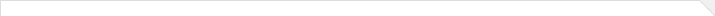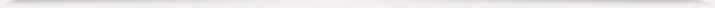报告题目： On Thurston's "geometric ideal triangulation" conjecture 报 告 人： 葛化彬 报告人所在单位： 中国人民大学 报告日期： 2022-02-23 报告时间： 10:30-11:30 报告地点： 光华楼东主楼 1601 报告摘要： Gluing ideal tetrahedra plays a crucial role in the construction of hyperbolic 3-manifolds. On the contrary, it is still not known that whether a hyperbolic 3-manifold admits a geometric ideal triangulation. One of the greatest achievement in three dimensional topology is the famous Hyperbolic Dehn filling Theorem. It was proved by Thurston at the end of the 1970s under the assumption that all cusped hyperbolic 3-manifolds admits a geometric triangulation. In this talk, we will show our program to hyperbolize and further obtain geometric triangulations of 3-manifolds. To be precise, we will show the connections between 3D-combinatorial Ricci flows and Thurston's geometric triangulations, that is, for a particular triangulation, the flow converges if and only if the triangulation is geometric. We will also give some topological conditions to guarantee the convergence of the combinatorial Ricci flows and furthermore the existence of geometric ideal triangulations. As far as we know, this is the first existence results for the geometric triangulations on a large class of 3-manifolds.学术海报.pdf 本年度学院报告总序号： 729